### Showing:

 Annotations Attributes Diagrams Instances Properties Source Used by
Element gml:ClothoidType / gml:refLocation
Namespace http://www.opengis.net/gml
Diagram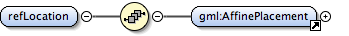Properties
 content: complex
Model gml:AffinePlacement
Children gml:AffinePlacement
Instance
 ``` {1,1} ```
Source
 ``` The "refLocation" is an affine mapping that places the curve defined by the Fresnel Integrals into the co-ordinate reference system of this object. ```
Schema location http://schemas.opengis.net/gml/3.1.1/base/geometryPrimitives.xsd
Element gml:ClothoidType / gml:scaleFactor
Namespace http://www.opengis.net/gml
Annotations
 ```The element gives the value for the constant in the Fresnel's integrals.```
Diagram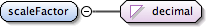Type decimal
Properties
 content: simple
Source
 ``` The element gives the value for the constant in the Fresnel's integrals. ```
Schema location http://schemas.opengis.net/gml/3.1.1/base/geometryPrimitives.xsd
Element gml:ClothoidType / gml:startParameter
Namespace http://www.opengis.net/gml
Annotations
 ```The startParameter is the arc length distance from the inflection point that will be the start point for this curve segment. This shall be lower limit used in the Fresnel integral and is the value of the constructive parameter of this curve segment at its start point. The startParameter can either be positive or negative. NOTE! If 0.0 (zero), lies between the startParameter and the endParameter of the clothoid, then the curve goes through the clothoid's inflection point, and the direction of its radius of curvature, given by the second derivative vector, changes sides with respect to the tangent vector. The term length distance for the```
Diagram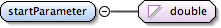Type double
Properties
 content: simple
Source
 ``` The startParameter is the arc length distance from the inflection point that will be the start point for this curve segment. This shall be lower limit used in the Fresnel integral and is the value of the constructive parameter of this curve segment at its start point. The startParameter can either be positive or negative. NOTE! If 0.0 (zero), lies between the startParameter and the endParameter of the clothoid, then the curve goes through the clothoid's inflection point, and the direction of its radius of curvature, given by the second derivative vector, changes sides with respect to the tangent vector. The term length distance for the ```
Schema location http://schemas.opengis.net/gml/3.1.1/base/geometryPrimitives.xsd
Element gml:ClothoidType / gml:endParameter
Namespace http://www.opengis.net/gml
Annotations
 ```The endParameter is the arc length distance from the inflection point that will be the end point for this curve segment. This shall be upper limit used in the Fresnel integral and is the value of the constructive parameter of this curve segment at its start point. The startParameter can either be positive or negative.```
Diagram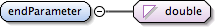Type double
Properties
 content: simple
Source
 ``` The endParameter is the arc length distance from the inflection point that will be the end point for this curve segment. This shall be upper limit used in the Fresnel integral and is the value of the constructive parameter of this curve segment at its start point. The startParameter can either be positive or negative. ```
Schema location http://schemas.opengis.net/gml/3.1.1/base/geometryPrimitives.xsd
Complex Type gml:ClothoidType
Namespace http://www.opengis.net/gml
Annotations
 ```A clothoid, or Cornu's spiral, is plane curve whose curvature is a fixed function of its length. In suitably chosen co-ordinates it is given by Fresnel's integrals. x(t) = 0-integral-t cos(AT*T/2)dT y(t) = 0-integral-t sin(AT*T/2)dT This geometry is mainly used as a transition curve between curves of type straight line to circular arc or circular arc to circular arc. With this curve type it is possible to achieve a C2-continous transition between the above mentioned curve types. One formula for the Clothoid is A*A = R*t where A is constant, R is the varying radius of curvature along the the curve and t is the length along and given in the Fresnel integrals.```
Diagram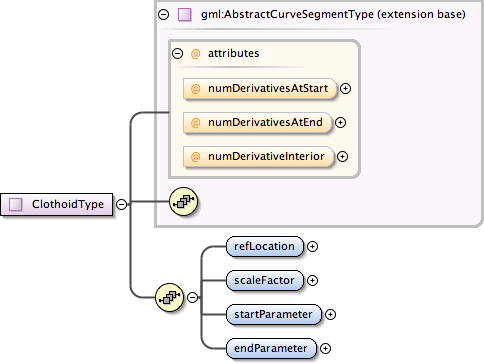Type extension of gml:AbstractCurveSegmentType
Type hierarchy
Used by
 Element gml:Clothoid
Model gml:refLocation , gml:scaleFactor , gml:startParameter , gml:endParameter
Children gml:endParameter, gml:refLocation, gml:scaleFactor, gml:startParameter
Attributes
QName Type Fixed Default Use Annotation
numDerivativeInterior integer 0 optional
 ```The attribute "numDerivativesInterior" specifies the type of continuity that is guaranteed interior to the curve. The default value of "0" means simple continuity, which is a mandatory minimum level of continuity. This level is referred to as "C 0 " in mathematical texts. A value of 1 means that the function and its first derivative are continuous at the appropriate end point: "C 1 " continuity. A value of "n" for any integer means the function and its first n derivatives are continuous: "C n " continuity. NOTE: Use of these values is only appropriate when the basic curve definition is an underdetermined system. For example, line string segments cannot support continuity above C 0 , since there is no spare control parameter to adjust the incoming angle at the end points of the segment. Spline functions on the other hand often have extra degrees of freedom on end segments that allow them to adjust the values of the derivatives to support C 1 or higher continuity.```
numDerivativesAtEnd integer 0 optional
 ```The attribute "numDerivativesAtEnd" specifies the type of continuity between this curve segment and its successor. If this is the last curve segment in the curve, one of these values, as appropriate, is ignored. The default value of "0" means simple continuity, which is a mandatory minimum level of continuity. This level is referred to as "C 0 " in mathematical texts. A value of 1 means that the function and its first derivative are continuous at the appropriate end point: "C 1 " continuity. A value of "n" for any integer means the function and its first n derivatives are continuous: "C n " continuity. NOTE: Use of these values is only appropriate when the basic curve definition is an underdetermined system. For example, line string segments cannot support continuity above C 0 , since there is no spare control parameter to adjust the incoming angle at the end points of the segment. Spline functions on the other hand often have extra degrees of freedom on end segments that allow them to adjust the values of the derivatives to support C 1 or higher continuity.```
numDerivativesAtStart integer 0 optional
 ```The attribute "numDerivativesAtStart" specifies the type of continuity between this curve segment and its predecessor. If this is the first curve segment in the curve, one of these values, as appropriate, is ignored. The default value of "0" means simple continuity, which is a mandatory minimum level of continuity. This level is referred to as "C 0 " in mathematical texts. A value of 1 means that the function and its first derivative are continuous at the appropriate end point: "C 1 " continuity. A value of "n" for any integer means the function and its first n derivatives are continuous: "C n " continuity. NOTE: Use of these values is only appropriate when the basic curve definition is an underdetermined system. For example, line string segments cannot support continuity above C 0 , since there is no spare control parameter to adjust the incoming angle at the end points of the segment. Spline functions on the other hand often have extra degrees of freedom on end segments that allow them to adjust the values of the derivatives to support C 1 or higher continuity.```
Source
 ``` A clothoid, or Cornu's spiral, is plane curve whose curvature is a fixed function of its length. In suitably chosen co-ordinates it is given by Fresnel's integrals. x(t) = 0-integral-t cos(AT*T/2)dT y(t) = 0-integral-t sin(AT*T/2)dT This geometry is mainly used as a transition curve between curves of type straight line to circular arc or circular arc to circular arc. With this curve type it is possible to achieve a C2-continous transition between the above mentioned curve types. One formula for the Clothoid is A*A = R*t where A is constant, R is the varying radius of curvature along the the curve and t is the length along and given in the Fresnel integrals. The "refLocation" is an affine mapping that places the curve defined by the Fresnel Integrals into the co-ordinate reference system of this object. The element gives the value for the constant in the Fresnel's integrals. The startParameter is the arc length distance from the inflection point that will be the start point for this curve segment. This shall be lower limit used in the Fresnel integral and is the value of the constructive parameter of this curve segment at its start point. The startParameter can either be positive or negative. NOTE! If 0.0 (zero), lies between the startParameter and the endParameter of the clothoid, then the curve goes through the clothoid's inflection point, and the direction of its radius of curvature, given by the second derivative vector, changes sides with respect to the tangent vector. The term length distance for the The endParameter is the arc length distance from the inflection point that will be the end point for this curve segment. This shall be upper limit used in the Fresnel integral and is the value of the constructive parameter of this curve segment at its start point. The startParameter can either be positive or negative. ```
Schema location http://schemas.opengis.net/gml/3.1.1/base/geometryPrimitives.xsd